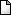# OpenCL Sorting

Eric Bainville - June 2011

## Introduction

Sorting algorithms are not the best suited for the GPU: they usually require lots of comparisons, and access memory through irregular patterns. However, since sorting is a basic building block of many algorithms, it may be desirable to have a GPU implementation. Some GPU implementations are actually faster than the CPU (assuming data is already resident in the GPU).

### Contents

2. Parallel selection - Parallel selection sort, some variations on a simple algorithm.
3. Parallel selection, local - Partial parallel selection sort inside a workgroup.
4. Parallel bitonic, local - Partial bitonic sort inside a workgroup.
5. Parallel bitonic I - Full bitonic sort, working in global memory and registers.
6. Parallel bitonic II - Full bitonic sort, adding local memory.
7. Parallel merge, local - Partial merge sort inside a workgroup.

### References

Writing this article was an excellent opportunity to re-open the third volume of the Art of Computer Programming by Donald E. Knuth. Googling for GPU sorting will return the most interesting references on the subject. The Wikipedia Sorting algorithm page provides some useful information too. Efficient algorithms for GPU sorting can be found in Designing Efficient Sorting Algorithms for Manycore GPUs by Satish, Harris, and Garland. The publications of Duane Merrill describe more recent high performance sorting on GPU (reaching 775 Mkey/s on a NVIDIA GTX 480 for Key+Value sorting).

The package contains all host and kernel code, with projects for Linux and Visual Studio 2010. It includes a small OpenCL wrapper providing basic useful functions (MiniCL). The test program will check all the kernels (it takes some time).OpenCLsorting-20110625.zip (24 KB), June 2011, all kernels up to Parallel Bitonic II.

### Benchmarks

We will sort an input array of N 32-bit unsigned integers keys, with and without an associated 32-bit integer value. For a given N we measure the elapsed wall clock time T, and performance P=10-6.N/T in Mkey/s (million keys per second). To simplify the code, N will always be a power of 2. The measurement loop repeats the sort until a minimal elapsed time is reached, as in the following:

```double t0 = getRealTime(); // wallclock time (s)
double dt = 0;
double nit = 0; // total number of calls to sort()
// Double number of iterations until we reach a minimum elapsed time (0.5s here)
for (int it=1;it<=1<<20;it<<=1)
{
for (int i=0;i<it;i++)
{
ok &= algo.sort(c,targetDevice,n,inBuffer,outBuffer); // sort
c->finish(targetDevice); // wait for kernels to terminate
}
dt = getRealTime() - t0; // elapsed time (s)
nit += (double)it;
if (dt > 0.5) break; // min time reached?
}
dt /= nit;  // elapsed time per algorithm invocation
```

The machine used for our experiments has the following configuration: Core i7 950 @4000MHz 12GB RAM, AMD HD6970 Cayman @880Mhz 2GB RAM @1375MHz, Windows 7 64-bit and AMD Catalyst 11.6.

### Upper bound of the sorting rate

To get an upper bound of the expected performance, let's imagine we will run K successive kernels, each run reading and writing the entire key array, (4+4).N bytes. Assuming we can reach the advertised 176 GB/s for the HD6970, we get rate < 22,000/K Mkey/s.